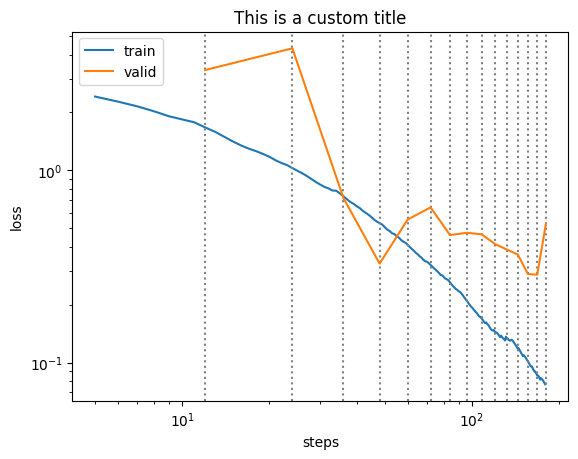# Improving plots, especially `plot_loss()`

Feature Request: I think that plots could be improved, especially the `recorder.plot_loss()` is missing a lot. If you agree, i will make an merge request.

Improves for `plot_loss()`:

• naming the axes
• adding vlines to indicate epochs
• possibility to plot logarithmic scale (as requested here)
• possibility to add an existing axis and return the axis for further customization*

*this should be standard for all plot functions. as many other libraries to this, for example darts

Improved code:

``````def plot_loss(self, skip_start=5, with_valid=True, log=False, show_epochs=False, ax=None):
if not ax:
ax=plt.gca()
if log:
ax.loglog(list(range(skip_start, len(self.losses))), self.losses[skip_start:], label='train')
else:
ax.plot(list(range(skip_start, len(self.losses))), self.losses[skip_start:], label='train')
if show_epochs:
for x in self.iters:
ax.axvline(x, color='grey', ls=':')
ax.set_ylabel('loss')
ax.set_xlabel('steps')
ax.set_title('learning curve')
if with_valid:
idx = (np.array(self.iters)<skip_start).sum()
valid_col = self.metric_names.index('valid_loss') - 1
ax.plot(self.iters[idx:], L(self.values[idx:]).itemgot(valid_col), label='valid')
ax.legend()
return ax
``````

Sample usage:

``````# example 1 (backwards compatible)
learn.recorder.plot_loss()

# example 2 (new features)
fig, ax = plt.subplots()
learn.recorder.plot_loss(log=True, show_epochs=True, ax=ax)
ax.set_title('This is a custom title') # further customization
``````

Example 1:Example 2:Issue: